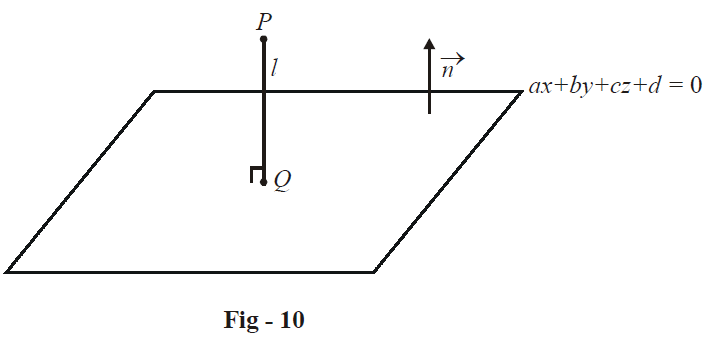# Examples on Equations of Three Dimensional Planes Set 2

Go back to  'Three Dimensional Geometry'

## Three dimensional planes

Example – 12

A plane is at a distance p from the origin and the direction cosines of the (outward) normal to it are l, m, n. Find its equation.

Solution: The unit vector  $$\hat n$$  normal to the plane is

$\hat n = l\hat i + m\hat j + n\hat k$

For any point  $$\vec r\left( {x\hat i + y\hat j + z\hat k} \right)$$  in the plane, we have

\begin{align}&\qquad\;\;\;\vec r \cdot \hat n = p \\\\ &\Rightarrow \quad lx + my + nz = p \\ \end{align}

This is the required equation; it is called the normal form of the plane’s equation. As an exercise, convert the general equation of the plane

$ax + by + cz + d = 0$

into normal form.

Example – 13

Find the angle of intersection of the two planes

\begin{align} {a_1}x + {b_1}y + {c_1}z + {d_1} = 0 \\\\ {a_2}x + {b_2}y + {c_2}z + {d_2} = 0 \\ \end{align}

Solution: From the equations of the planes, it is evident that the following vectors are to these planes arespectively:

\begin{align} {{\vec n}_1} = {a_1}\hat i + {b_1}\hat j + {c_1}\hat k \\\\ {{\vec n}_2} = {a_2}\hat i + {b_2}\hat j + {c_2}\hat k \\ \end{align}

Since the acute angle  $$\theta$$ between the two planes will be the acute angle between their normals, we have

\begin{align}&\cos \theta = \frac{{\left| {{{\vec n}_1} \cdot {{\vec n}_2}} \right|}}{{\left| {{{\vec n}_1}} \right|\left| {{{\vec n}_2}} \right|}} \\\\ \,\,\,\,\,\,\,\,\,\,\, &\qquad= \frac{{\left| {{a_1}{a_2} + {b_1}{b_2} + {c_1}{c_2}} \right|}}{{\sqrt {a_1^2 + b_1^2 + c_1^2} \sqrt {a_2^2 + b_2^2 + c_2^2} }} \\ \end{align}

Incidentally, we can now derive the conditions for these planes to be parallel or perpendicular.

Planes are parallel if $${\vec n_1} = \lambda {\vec n_2}$$        \begin{align} \Rightarrow \,\,\,\,\,\,\,\,\,\frac{{{a_1}}}{{{a_2}}} = \frac{{{b_1}}}{{{b_2}}} = \frac{{{c_1}}}{{{c_2}}}\end{align}

Planes are perpendicular if  $${\vec n_1} \times {\vec n_2} = 0$$         $$\Rightarrow \,\,\,\,\,\,\,\,\,\,\,\,\,{a_1}{a_2} + {b_1}{b_2} + {c_1}{c_2} = 0$$

It should be obvious that for two parallel planes, their equations can be written so that they differ only in the constant term. Thus, any plane parallel to  $$ax + by + cz + d = 0$$  can be written as  $$ax + by + cz + d' = 0$$ where   $$d' \in \mathbb{R}\,\,\left( {{\text{and }}d' \ne d} \right).$$

Example – 14

(a) Find the distance of the point  $$P\left( {{x_1},{y_1},{z_1}} \right)$$  from the plane  $$ax + by + cz + d = 0.$$

(b) Find the distance between the two parallel planes

\begin{align} ax + by + cz + {d_1} = 0 \\\\ ax + by + cz + {d_2} = 0 \\\end{align}

Solution:(a) The distance l of P from the given plane will obviously be measured along the normal to the plane passing through P:We write the equation of the plane as

$\vec r \cdot \vec n = - d$

where  $$\vec r = x\hat i + y\hat j + z\hat k$$  is any point on the plane and  $$\vec n = a\hat i + b\hat j + c\hat k$$  is the normal to the plane. Let O be the origin. Since Q lies on the plane, its position vector $$\overrightarrow {OQ}$$  must satisfy the equation of the plane. But  $$\overrightarrow {OQ} = \overrightarrow {OP} + \overrightarrow {PQ} .$$    Thus,

$\left( {\overrightarrow {OP} + \overrightarrow {PQ} } \right) \cdot \vec n = - d$

Note that  $$\overrightarrow {PQ} = \frac{{\lambda \vec n}}{{\left| {\vec n} \right|}}$$   where   $$\lambda = \pm l$$  (which sign to take depends on which direction  $$\vec n$$  points in).

Thus,

\begin{align}&\qquad\;\;\;\;\left( {\overrightarrow {OP} + \frac{{\lambda \vec n}}{{\left| {\vec n} \right|}}} \right) \cdot \vec n = - d \\\\ &\Rightarrow \quad \overrightarrow {OP} \cdot \vec n + \frac{{\lambda \vec n \cdot \vec n}}{{\left| {\vec n} \right|}} = - d \\\\ &\Rightarrow \quad\left( {{x_1}\hat i + {y_1}\hat j + {z_1}\hat k} \right) \cdot \left( {a\hat i + b\hat j + c\hat k} \right) + \lambda \left| {\vec n} \right| = - d \\\\ &\Rightarrow \quad a{x_1} + b{y_1} + c{z_1} + d = - \lambda \left| {\vec n} \right| \\ &\Rightarrow \quad \left| \lambda \right| = l = \frac{{\left| {a{x_1} + b{y_1} + c{z_1} + d} \right|}}{{\left| {\vec n} \right|}} \\ \\ &\Rightarrow \quad l = \frac{{\left| {a{x_1} + b{y_1} + c{z_1} + d} \right|}}{{\sqrt {{a^2} + {b^2} + {c^2}} }} \\ \end{align}

(b) Assume any point  $$P\left( {{x_1},{y_1},{z_1}} \right)$$ on the first plane. We have

\begin{align}&\qquad\;\;\; a{x_1} + b{y_1} + c{z_1} + {d_1} = 0 \\\\ &\Rightarrow \quad {d_1} = - \left( {a{x_1} + b{y_1} + c{z_1}} \right)\,\,\,\,\,\,\,\,\,\,\,\,\,\,\,\,\,\,\,\,\,\,\,\,\,\,\, \ldots \left( 1 \right) \\ \end{align}

The distance of P from the second plane, say l, can be evaluated as described in part (a) above :

$l = \frac{{\left| {a{x_1} + b{y_1} + c{z_1} + {d_2}} \right|}}{{\sqrt {{a^2} + {b^2} + {c^2}} }}\,\,\,\,\,\,\,\,\,\,\,\,\,\,\,\,\,\,\,\,\,\,\,\,\,\,\,\,\, \ldots \left( 2 \right)$

Using (1) in (2), we have

$l = \frac{{\left| {{d_2} - {d_1}} \right|}}{{\sqrt {{a^2} + {b^2} + {c^2}} }}$

This is the required distance between the two planes.

Learn from the best math teachers and top your exams

• Live one on one classroom and doubt clearing
• Practice worksheets in and after class for conceptual clarity
• Personalized curriculum to keep up with school